# Why Is Your Excel Formula Not Calculating?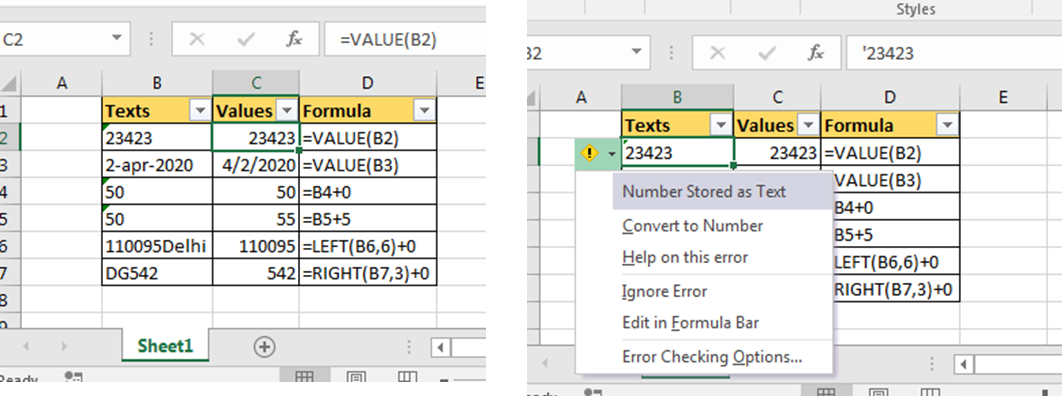Scenario:

Many times, like when we extract numbers out of string, they are text by property. You can’t do any number operations on them. Then we get the need of converting that text to a number. So to convert any text to a number we can take various approaches depending on the situation. Let’s have a look at those approaches…

#### Use VALUE Function to Convert Text into Number

So if you downloaded sum data from a website, it's common to get some numbers as text format special dates. Just wrap these text into a VALUE function. It will return a pure number.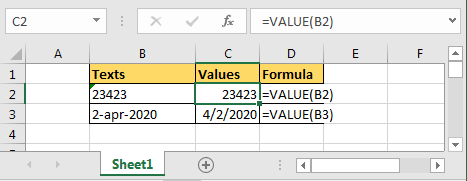VALUE function Syntax

 =VALUE(text)

So to convert a text in cell B2 into number write this VALUE formula.

 =VALUE(B2)

#### Add 0 to text to Convert Text into Number

If you add any number using + operator to a text formatted number, it will convert the text to a number and then add the given number to the converted number. Finally the result will be a number.

For example if there is a text formatted number in B4, then just add 0 to convert string to number.

 =B4+0

Result will be a number for sure.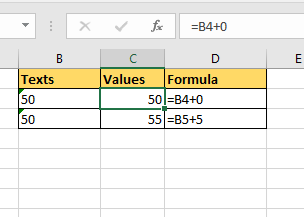As I told you in the beginning, this problem occurs when we extract numbers from strings. So if you want the extracted text to be numbered just add 0 in the end.

For example I am extracting citycode from text B6. I extracted it using the LEFT function. But it's not a number so I added a 0 in the formula itself.

 =LEFT(B6,6)+0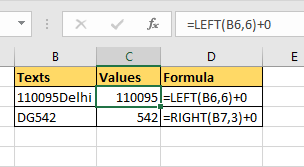#### Using Excel Notification to Convert Text into Number

Whenever a number is in the form of a text, excel notifies you by showing a green corner of the cell.

When you click on the cell it shows a small exclamation icon at the left corner of the cell.

When you click on it, it shows an option of Convert to Number. Click on it and the text will be converted to number.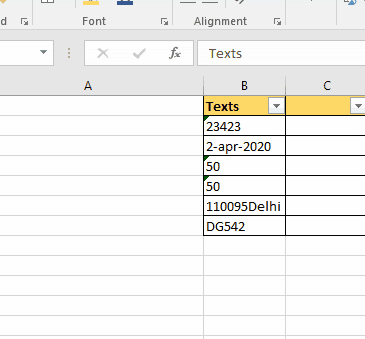Convert Text to Number using VBA
So, if you have a fixed range that you want to convert to text then use this VBA snippet.

```Sub ConvertTextToNumber()
Range("A3:A8").NumberFormat = "General"
Range("A3:A8").Value = Range("A3:A8").Value
End Sub

```

When you run the above code it converts the text of range A3:A8 into text.
This code can look more elegant if we write it like this.

```Sub ConvertTextToNumber()

With Range("A3:A8")
.NumberFormat = "General"
.Value = .Value
End With

End Sub

```

How does it work?

Well, it is quite simple. We first change the number format of the range to General. Then we put the value of that range into the same range using VBA. This removes the text formatting completely. Simple, isn't it?

Change the number of formatting of a dynamic range

In the above code, we changed the text to number in the above code of a fixed range but this will not be the case most of the time. To convert text to a number of dynamic ranges, we can evaluate the last used cell or select the range dynamically.

This is how it would look:

```Sub ConvertTextToNumber()
With Range("A3:A" & Cells(Rows.Count, 1).End(xlUp).Row)
.NumberFormat = "General"
.Value = .Value
End With
```

Here, I know that the range starts from A3. But I don't know where it may end.

So I dynamically identify the last used excel row that has data in it using the VBA snippet Cells(Rows.Count, 1).End(xlUp).Row. It returns the last used row number that we are concatenating with "A3:A".

Note: This VBA snippet will work on the active workbook and active worksheet. If your code switches through multiple sheets, it would be better to set a range object of the intended worksheet. Like this

```Sub ConvertTextToNumber()
Set Rng = ThisWorkbook.Sheets("Sheet1").Range("A3:A" & Cells(Rows.Count, 1).End(xlUp).Row)
With Rng
.NumberFormat = "General"
.Value = .Value
End With
End Sub

```

The above code will always change the text to the number of the sheet1 of the workbook that contains this code.

#### Loop and CSng to change the text to number

Another method is to loop through each cell and change the cell value to a number using the CSng function. Here's the code.

```Sub ConvertTextToNumberLoop()
Set Rng = ThisWorkbook.Sheets("Sheet1").Range("A3:A" & Cells(Rows.Count, 1).End(xlUp).Row)

For Each cel In Rng.Cells
cel.Value = CSng(cel.Value)
Next cel

End Sub
```

In the above VBA snippet, we are using VBA For loop to iterate over each cell in the range and convert the value of each cell into a number using the CSng function of VBA.

So yeah guys, this is how you can change texts to numbers in Excel using VBA. You can use these snippets to ready your worksheet before you do any number operation on them. I hope I was explanatory enough. You can download the working file here.

Hope this article about Why Is Your Excel Formula Not Calculating is explanatory. Find more articles on calculating values and related Excel formulas here. If you liked our blogs, share it with your friends on Facebook. And also you can follow us on Twitter and Facebook. We would love to hear from you, do let us know how we can improve, complement or innovate our work and make it better for you. Write to us at info@exceltip.com.

Related Articles :

How to get Text & Number in Reverse through VBA in Microsoft Excel : To reverse number and text we use loops and mid function in VBA. 1234 will be converted to 4321, "you" will be converted to "uoy". Here's the snippet.

Format data with custom number formats using VBA in Microsoft Excel : To change the number format of specific columns in excel use this VBA snippet. It converts the number format of specified to specified format in one click.

Popular Articles :

How to use the IF Function in Excel : The IF statement in Excel checks the condition and returns a specific value if the condition is TRUE or returns another specific value if FALSE.

How to use the VLOOKUP Function in Excel : This is one of the most used and popular functions of excel that is used to lookup value from different ranges and sheets.

How to use the SUMIF Function in Excel : This is another dashboard essential function. This helps you sum up values on specific conditions.

How to use the COUNTIF Function in Excel : Count values with conditions using this amazing function. You don't need to filter your data to count specific values. Countif function is essential to prepare your dashboard.

1.